# GSEB Solutions Class 7 Maths Chapter 14 Symmetry Ex 14.1

Gujarat Board GSEB Textbook Solutions Class 7 Maths Chapter 14 Symmetry Ex 14.1 Textbook Questions and Answers.

## Gujarat Board Textbook Solutions Class 7 Maths Chapter 14 Symmetry Ex 14.1Question 1.
Copy the figures with punched holes and find the axes of symmetry for the following:Solution:
The axes of symmetry are shown by the dotted lines in the following figures: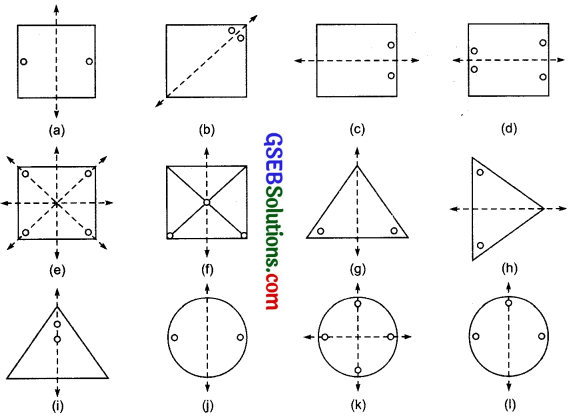Question 2.
Given the line(s) of symmetry, find the other hole(s):Solution:
The figures with other holes are given below:Question 3.
In the following figures, the mirror line (i.e. the line of symmetry) is given as a dotted line. Complete each figure performing reflection in the dotted (mirror) line. (You might perhaps place a mirror along the dotted line and look into the mirror for the image.) Are you able to recall the name of the figure you complete?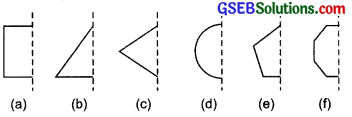Solution:
The completed figures (i.e. the reflected figures) are given below along with the name of the figure: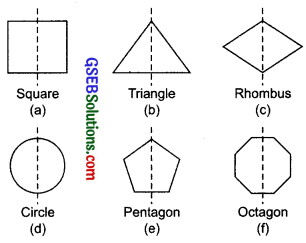Question 4.
The following figures have more than one line of symmetry. Such figures are said to have multiple lines of symmetry.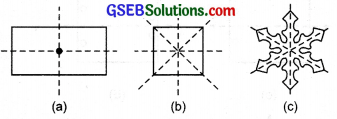Identify multiple lines of symmetry, if any, in each of the following figures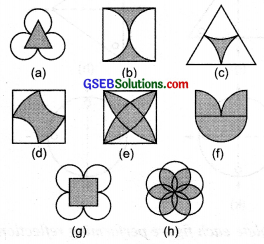Question 5.
Copy the figure given here. Take any one diagonal as a line of symmetry and shade a few more squares to make the figure symmetric about a diagonal. Is there more than one way to do that? Will the figure be symmetric about both the diagonals?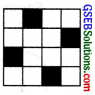Solution:
(i) Let us take the diagonal AC and shade the squares as shown in the figure. The figure is symmetric about AC.
(ii) Since, the figure is symmetric about EF and GH also. Thus there are more than one way to make it symmetric.
(iii) The figure is symmetric ® about the diagonal BD also.∴ The figure is symmetric about both the diagonals.Question 6.
Copy the diagram and complete each shape to be symmetric about the mirror line(s):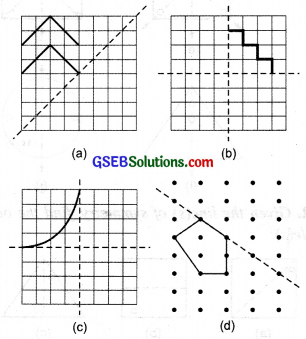Solution: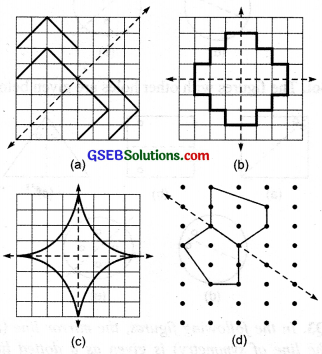Question 7.
State the number of lines of symmetry for the following figures:
(a) An equilateral triangle
(b)An isosceles triangle
(c) A scalene triangle
(d) A square
(e) A rectangle
(f) A rhombus
(g) A parallelogram
(i) A regular hexagon
(j) A circle
Solution:
(a) An equilateral triangle has 3 lines of symmetry.
(b) An isosceles triangle has 1 line of symmetry.
(c) A scalene triangle has 0 line of symmetry.
(d) A square has 4 lines of symmetry.
(e) A rectangle has 2 lines of symmetry.
(f) A rhombus has 4 lines of symmetry.
(g) A parallelogram has 0 line of symmetry.
(h) A quadrilateral has 0 line of symmetry.
(i) A regular hexagon has 6 lines of symmetry.
(j) A circle has infinitely many lines of symmetry.Question 8.
What letters of the English alphabet have reflectional symmetry (i.e. symmetry related to mirror reflection) about
(a) a vertical mirror
(b) a horizontal mirror
(c) both horizontal and vertical mirrors
Solution:
(a) Letters of the English alphabet having reflectional symmetry about a vertical mirror are:
A, H, I, M, O, T, U, V, W, X and Y

(b) Letters of the English alphabet having reflectional symmetry about a horizontal mirror are:
B, C, D, E, H, I, O and X

(c) Letters of the English alphabet having reflectional symmetry about both horizontal and vertical mirrors are:
O, X, I and H

Question 9.
Give three examples of shapes with no line of symmetry.
Solution:
(i) A scalene triangle
(ii) The letter F
(iii) A parallelogramQuestion 10.
What other name can you give to the line of symmetry of
(a) an isosceles triangle?
(b) a circle?
Solution:
(a) Median
(b) Diameter.